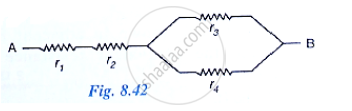# Calculate the Equivalent Resistance of the Following Combination of Resistors R1, R2, R3 and R4 If R_1=R_2=R_3=R_4=2.0ω  Between the Points a and B in Fig. 8.42 - Physics

Calculate the equivalent resistance of the following combination of resistors r1, r2, r3 and r4 if r_1=r_2=r_3=r_4=2.0Ω  between the points A and B in Fig. 8.42#### Solution

r_1=r_2=r_3=r_4=2.0ohm

r=r_1+r_2=2+2=4ohm

1/r=1/r_3+1/r_4=1/2+1/2=1

r=1ohm

r=r+r=4+1=5ohm

Concept: System of Resistors - Resistances in Parallel
Is there an error in this question or solution?

#### APPEARS IN

Selina Concise Physics Class 10 ICSE
Chapter 8 Current Electricity
Exercise 8 (B) | Q 10 | Page 201